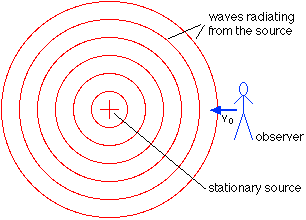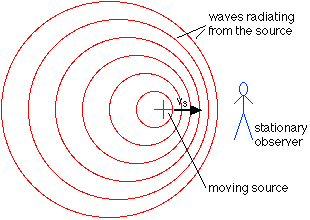# Doppler equation not making sense to me

## Homework Statement

So lets say a source is moving towards an observer at a speed of .9c where c is the speed of sound. the original frequency is 1 Hz.

final frequency = (v(sound) / ((v(sound)-v(source))) * initial frequency
so the final frequency would increase by a factor of 10.

Now lets say that the observer was moving towards the source.

final frequency = (v(sound) + v(observer))/ (v(sound)))intial frequency
where in this case the final freuqnecy would only increase by a factor of 1.9Hz.

Why am I getting different answers? Why does it matter whether the source is moving towards the observer or the observer towards the source?

D H
Staff Emeritus
Why does it matter whether the source is moving towards the observer or the observer towards the source?
It's because something quite different is happening depending on whether the source or receiver is moving. From http://physics.bu.edu/~duffy/py105/Doppler.html:Moving source:•1 person
BruceW
Homework Helper
Why am I getting different answers? Why does it matter whether the source is moving towards the observer or the observer towards the source?
The key is to think about the medium through which the wave is moving. For light, there is no medium, so in that case, you would get the same answer in either case. But sound does have a medium.

•1 person
D H
Staff Emeritus
Side note: The Doppler effect for light does not exhibit this different behavior depending on whether it's the source or receiver that is moving. You can't even say which one is moving, which one is stationary with light. Moving / stationary with respect to what?

You can say which one is moving, which one is stationary with sound. Sound needs a medium. The speed is measured with respect to the medium, the air in this case. Light doesn't need a medium. There is no luminiferous aether.

Edit: Bruce beat me to it.

•1 person
BruceW
Homework Helper
hehe, by the skin of my teeth.